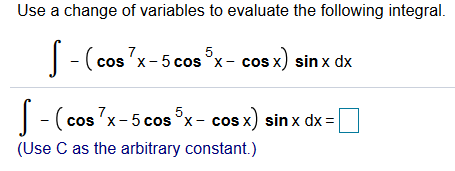# Use a change of variables to evaluate the following integralcos x) sin x dx5-(cos 'x-5 cos x-c(cos 7x-5 cos x- cos x) sinx dx5(Use C as the arbitrary constant.)

Question
1 viewshelp_outlineImage TranscriptioncloseUse a change of variables to evaluate the following integral cos x) sin x dx 5 -(cos 'x-5 cos x-c (cos 7x-5 cos x- cos x) sinx dx 5 (Use C as the arbitrary constant.) fullscreen
check_circle

Step 1

The given integral is,

Step 2

The above integral can be split into the following integrals.

Step 3

The first integral is evaluated using...

### Want to see the full answer?

See Solution

#### Want to see this answer and more?

Solutions are written by subject experts who are available 24/7. Questions are typically answered within 1 hour.*

See Solution
*Response times may vary by subject and question.
Tagged in

### Integration